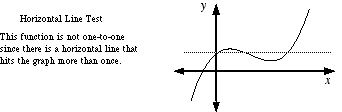index: click on a letter A B C D E F G H I J K L M N O P Q R S T U V W X Y Z A to Z index index: subject areas numbers & symbols sets, logic, proofs geometry algebra trigonometry advanced algebra & pre-calculus calculus advanced topics probability & statistics real world applications multimedia entrieswww.mathwords.com about mathwords website feedback

 Horizontal Line Test A test use to determine if a function is one-to-one. If a horizontal line intersects a function's graph more than once, then the function is not one-to-one. Note: The function y = f(x) is a function if it passes the vertical line test. It is a one-to-one function if it passes both the vertical line test and the horizontal line test.